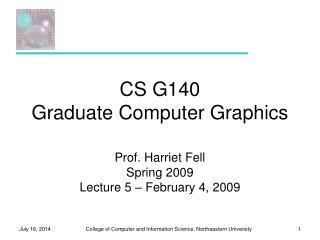Download Presentation# CS G140 Graduate Computer Graphics - PowerPoint PPT PresentationDownload Presentation## CS G140 Graduate Computer Graphics

- - - - - - - - - - - - - - - - - - - - - - - - - - - E N D - - - - - - - - - - - - - - - - - - - - - - - - - - -
##### Presentation Transcript

1. CS G140Graduate Computer Graphics Prof. Harriet Fell Spring 2009 Lecture 5 – February 4, 2009

2. Comments • “NOTHING else” means nothing else. • Do you want your pictures on the web? • If not, please send me an email.

3. Today’s Topics • Bump Maps • Texture Maps --------------------------- • 2D-Viewport Clipping • Cohen-Sutherland • Liang-Barsky

4. v u Bump Maps - Blinn 1978 Surface P(u, v) N

5. One dimensional Example P(u) B(u)

6. The New Surface B(u) P’(u)= P(u) + B(u)N

7. Bump Maps - Formulas

8. The New Normal This term is 0. These terms are small if B(u, v) is small.

9. Tweaking the Normal Vector D lies in the tangent plane to the surface.

10. Plane with Spheres Plane with Horizontal Wave

11. Plane with Vertical Wave Plane with Ripple

12. Plane with Mesh Plane with Waffle

13. Plane with Dimples Plane with Squares

14. Dots and Dimples

15. Plane with Ripples

16. Sphere on Plane with Spheres Sphere on Plane with Horizontal Wave

17. Sphere on Plane with Vertical Wave Sphere on Plane with Ripple

18. Sphere on Plane with Mesh Sphere on Plane with Waffle

19. Sphere on Plane with Dimples Sphere on Plane with Squares

20. Sphere on Plane with Ripples

21. Wave with Spheres Parabola with Spheres

22. Parabola with Dimples Big Sphere with Dimples

23. Parabola with Squares Big Sphere with Squares

24. Big Sphere with Vertical Wave Big Sphere with Mesh

25. Cone Vertical with Wave Cone with Dimples

26. Cone with Ripple Cone with Ripples

27. Student Images

28. Bump Map - Plane x = h - 200; y = v - 200; z = 0; N.Set(0, 0, 1); Du.Set(-1, 0, 0); Dv.Set(0, 1, 0); uu = h; vv = v; zz = z;

29. Bump Map Code – Big Sphere radius = 280.0; z = sqrt(radius*radius - y*y - x*x); N.Set(x, y, z); N = Norm(N); Du.Set(z, 0, -x); Du = -1*Norm(Du); Dv.Set(-x*y, x*x +z*z, -y*z); Dv = -1*Norm(Dv); vv = acos(y/radius)*360/pi; uu = (pi/2 +atan(x/z))*360/pi; zz = z;

30. Bump Map Code – Dimples Bu = 0; Bv = 0; iu = (int)uu % 30 - 15; iv = (int)vv % 30 - 15; r2 = 225.0 - (double)iu*iu - (double)iv*iv; if (r2 > 100) { if (iu == 0) Bu = 0; else Bu = (iu)/sqrt(r2); if (iv == 0) Bv = 0; else Bv = (iv)/sqrt(r2); }

31. Image as a Bump Map A bump map is a gray scale image; any image will do. The lighter areas are rendered as raised portions of the surface and darker areas are rendered as depressions. The bumping is sensitive to the direction of light sources. http://www.cadcourse.com/winston/BumpMaps.html

32. Time for a Break

33. Bump Map from an ImageVictor Ortenberg

34. Simple Textures on Planes Parallel to Coordinate Planes

35. Stripes

36. Checks

37. Stripes and Checks Red and Blue Stripes if ((x % 50) < 25) color = red else color = blue 0 24 49 Cyan and Magenta Checks if (((x % 50) < 25 && (y % 50) < 25)) || (((x % 50) >= 25 && (y % 50) >= 25))) color = cyan else color = magenta What happens when you cross x = 0 or y = 0?

38. Stripes, Checks, Image

39. Mona Scroll

40. Textures on 2 Planes

41. Mapping a Picture to a Plane • Use an image in a ppm file. • Read the image into an array of RGB values. Color myImage[width][height] • For a point on the plane (x, y, d) theColor(x, y, d) = myImage(x % width, y % height) • How do you stretch a small image onto a large planar area?

42. Other planes and Triangles N Given a normal and 2 points on the plane: Make u from the two points. v = N x u Express P on the plane as P = P0+ au + bv. P1 N x u = v u P0

43. Mandrill Sphere

44. Mona Spheres

45. Tova Sphere

46. More Textured Spheres